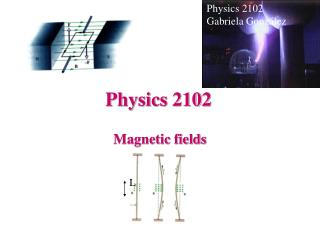DownloadDownload PresentationPhysics 2102

# Physics 2102

Download Presentation## Physics 2102

- - - - - - - - - - - - - - - - - - - - - - - - - - - E N D - - - - - - - - - - - - - - - - - - - - - - - - - - -
##### Presentation Transcript

1. Physics 2102 Gabriela González L Physics 2102 Magnetic fields

2. L Magnetic force on a wire Note: If wire is not straight, compute force on differential elements and integrate:

3. L R R L Example Wire with current i. Magnetic field out of page. What is net force on wire? By symmetry, F2 will only have a vertical component, Notice that the force is the same as that for a straight wire, and this would be true no matter what the shape of the central segment!.

4. Torque on a current loop: Principle behind electric motors. Rectangular coil: a x b, current = i Net force on current loop = 0 But: Net torque is NOT zero! • For a coil with N turns, • = N I A B sinq, where A is the area of coil

5. Right hand rule: curl fingers in direction of current; thumb points along m Define: magnetic dipole moment m Magnetic Dipole Moment We just showed: t = NiABsinq N=number of turns in coil A=area of coil. As in the case of electric dipoles, magnetic dipoles tend to align with the magnetic field (where potential energy is a minimum).

6. +Q p=Qa -Q QE q QE Electric and magnetic dipoles

7. Magnetic forces: summary • Magnetic force on a moving electric charge:F = qvxB • Magnetic Force on a wire:F = iLxB • Magnetic Torque on a magnetic dipole: t = mxB (potential energy: U= -m·B )

8. Example A magnetic dipole with a dipole moment of magnitude 0.02 J/T is released from rest in a uniform magnetic field of magnitude 50 mT. The rotation of the dipole due to the magnetic force on it is unimpeded. When the dipole rotates through the orientation where its dipole moment is aligned with the magnetic field, its kinetic energy is 0.70 mJ. (a) What is the initial angle between the dipole moment and the magnetic field?(b) What is the angle when the dipole is next (momentarily) at rest?

9. Example A wire of 62.0 cm length and 13 g mass is suspended by a pair of flexible leads in a magnetic field of 0.440 T. What are the magnitude and direction of the current required to remove the tension in the supporting leads?

10. Magnetic fields • Electric fields are created: • microscopically, by electric charges (fields) of elementary particles (electrons, protons) • macroscopically, by adding the field of many elementary charges of the same sign • Magnetic fields are created : • microscopically, by magnetic “moments” of elementary particles (electrons, protons, neutrons) • macroscopically, by • adding many microscopic magnetic moments (magnetic materials); or by • electric charges that move (electric currents)

11. Which we can write as, Currents producing magnetic fields:Biot-Savart Law When we computed the electric field due to charges we used Coulomb’s law. If one had a large irregular object, one broke it into infinitesimal pieces and computed, Magnetic fields are produced by electrical currents. If you wish to compute the magnetic field due to a current in a wire, you use the law of Biot and Savart.

12. ? Compare with: The Biot-Savart Law Jean-Baptiste Biot (1774-1862) Felix Savart (1791-1841) i • Quantitative rule for computing the magnetic field from any electric current • Choose a differential element of wire of length dL and carrying a current i • The field dB from this element at a point located by the vector r is given by the Biot-Savart Law: m0 =4px10-7 T.m/A (permeability constant)